# Import Text Files Into Numpy Arrays

• Difficulty Level : Medium
• Last Updated : 16 Mar, 2021

Numpy is an acronym for ‘Numerical Python’. It is a library in python for supporting n-dimensional arrays. But have you ever wondered about loading data into NumPy from text files. Don’t worry we will discuss the same in this article. To import Text files into Numpy Arrays, we have two functions in Numpy:

1. numpy.loadtxt( ) – Used to load text file data
2. numpy.genfromtxt( ) – Used to load data from a text file, with missing values handled as defined.

Note: numpy.loadtxt( ) is equivalent function to numpy.genfromtxt( ) when no data is missing.

Method 1 : numpy.loadtxt()

Syntax :

numpy.loadtxt(fname, dtype = float, comments=’#’, delimiter=None, converters=None, skiprows=0, usecols=None, unpack=False, ndmin=0, encoding=’bytes’, max_rows=None, *, like= None)

The default data type(dtype) parameter for numpy.loadtxt( ) is float.

Example 1: Importing Text file into Numpy arrays

The following ‘example1.txt’ text file is considered in this example.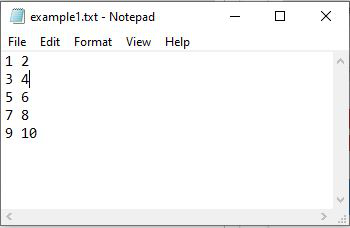## Python3

 `import` `numpy as np`` ` ` ` `# Text file data converted to integer data type``File_data ``=` `np.loadtxt(``"example1.txt"``, dtype``=``int``)``print``(File_data)`

Output :

```[[ 1  2]
[ 3  4]
[ 5  6]
[ 7  8]
[ 9 10]]```

Example 2: Importing text file into NumPy array by skipping first row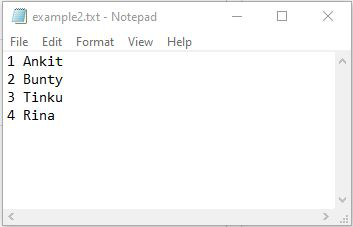## Python3

 `import` `numpy as np`` ` ` ` `# skipping first row``# converting file data to string``data ``=` `np.loadtxt(``"example2.txt"``, skiprows``=``1``, dtype``=``'str'``)``print``(data)`

Output :

```[['2' 'Bunty']
['3' 'Tinku']
['4' 'Rina']]```

Example 3: Importing only the first column(Names) of text file into numpy arrays

The indexing in NumPy arrays starts from 0. Hence, the Roll column in the text file is the 0th column, Names column is the 1st column and the Marks are the 2nd column in the text file ‘example3.txt’.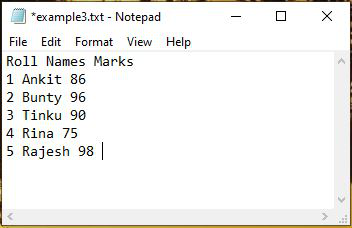## Python3

 `import` `numpy as np`` ` ` ` `# only column1 data is imported into numpy``# array from text file``data ``=` `np.loadtxt(``"example3.txt"``, usecols``=``1``, skiprows``=``1``, dtype``=``'str'``)`` ` `for` `each ``in` `data:``    ``print``(each)`

Output :

```Ankit
Bunty
Tinku
Rina
Rajesh```

Method 2 : numpy.genfromtxt()

Syntax :

numpy.genfromtxt(fname, dtype=float, comments=’#’, delimiter=None, skip_header=0, skip_footer=0, converters=None, missing_values=None, filling_values=None, usecols=None, names=None,excludelist=None, deletechars=” !#\$%&'()*+, -./:;<=>?@[\\]^{|}~”, replace_space=’_’, autostrip=False, case_sensitive=True, defaultfmt=’f%i’, unpack=None, usemask=False, loose=True, invalid_raise=True, max_rows=None, encoding=’bytes’, *, like=None)

Except the fname(filename) in numpy.genfromtxt( ), all the other parameters are optional.

Example 1: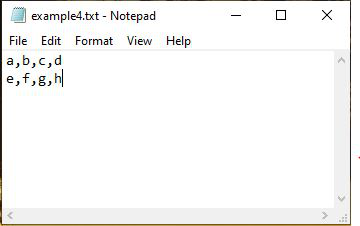## Python3

 `import` `numpy as np`` ` `Data ``=` `np.genfromtxt(``"example4.txt"``, dtype``=``str``,``                     ``encoding``=``None``, delimiter``=``","``)``print``(Data)`

Output :

```[['a' 'b' 'c' 'd']
['e' 'f' 'g' 'h']]```

Example 2: Importing text file into numpy arrays by skipping last row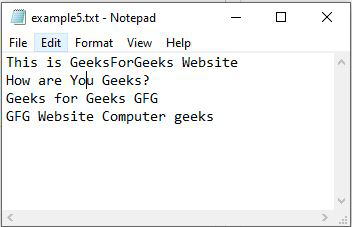## Python3

 `import` `numpy as np`` ` ` ` `# skipping last line in the file``Data ``=` `np.genfromtxt(``"example5.txt"``, dtype``=``str``,``                     ``encoding``=``None``, skip_footer``=``1``)``print``(Data)`

Output :

```[['This' 'is' 'GeeksForGeeks' 'Website']
['How' 'are' 'You' 'Geeks?']
['Geeks' 'for' 'Geeks' 'GFG']]```

My Personal Notes arrow_drop_up